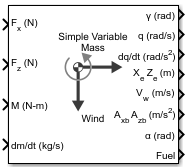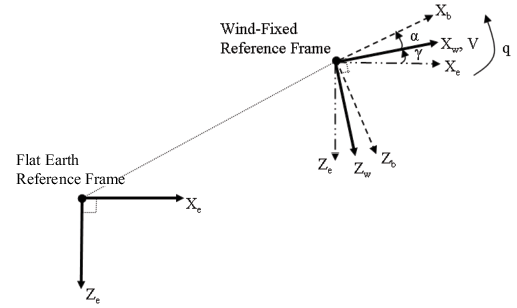# Simple Variable Mass 3DOF (Wind Axes)

Implement three-degrees-of-freedom equations of motion of simple variable mass with respect to wind axes

• Library:
• Aerospace Blockset / Equations of Motion / 3DOF

•## Description

The Simple Variable Mass 3DOF (Wind Axes) block implements three-degrees-of-freedom equations of motion of simple variable mass with respect to wind axes. The block considers the rotation in the vertical plane of a wind-fixed coordinate frame about a flat Earth reference frame. For more information about the rotation and equations of motion, see Algorithms.

## Ports

### Input

expand all

Applied force along the wind x-axis, specified as a scalar, in the units selected in Units.

Data Types: `double`

Applied force along the wind z-axis, specified as a scalar.

Data Types: `double`

Applied pitching moment, specified as a scalar.

Data Types: `double`

Rate of change of mass (positive if accreted, negative if ablated), specified as a scalar.

Data Types: `double`

Gravity, specified as a scalar.

#### Dependencies

To enable this port, set Gravity source to `External`.

Data Types: `double`

Relative velocity at which mass is accreted to or ablated from the body in body-fixed axes, specified as a two-element vector.

#### Dependencies

To enable this port, select Include mass flow relative velocity.

Data Types: `double`

### Output

expand all

Flight path angle, within ±pi, returned as a scalar, in radians.

Data Types: `double`

Pitch angular rate, returned as a scalar, in radians per second.

Data Types: `double`

Pitch angular acceleration, returned as a scalar, in radians per second squared.

Data Types: `double`

Location of the body in the flat Earth reference frame, (Xe, Ze), returned as a two-element vector.

Data Types: `double`

Velocity of the body resolved into the wind-fixed coordinate frame, (V, 0), returned as a two-element vector.

Data Types: `double`

Acceleration of the body with respect to the body-fixed coordinate frame, (Ax, Az), returned as a two-element vector.

Data Types: `double`

Angle of attack, returned as a scalar, in radians.

Data Types: `double`

Fuel tank status, returned as:

• `1` — Tank is full.

• `0` — Tank is neither full nor empty.

• `-1` — Tank is empty.

#### Dependencies

To enable this port, set Mass type to `Simple Variable`.

Data Types: `double`

Accelerations of the body with respect to the inertial (flat Earth) coordinate frame, returned as a two-element vector. You typically connect this signal to the accelerometer.

#### Dependencies

To enable this port, select the Include inertial acceleration check box.

Data Types: `double`

## Parameters

expand all

### Main

Input and output units, specified as `Metric (MKS)`, `English (Velocity in ft/s)`, or `English (Velocity in kts)`.

UnitsForcesMomentAccelerationVelocityPositionMassInertia
`Metric (MKS)` NewtonNewton-meterMeters per second squaredMeters per secondMetersKilogramKilogram meter squared
`English (Velocity in ft/s)` PoundFoot-poundFeet per second squaredFeet per secondFeetSlugSlug foot squared
`English (Velocity in kts)` PoundFoot-poundFeet per second squaredKnotsFeetSlugSlug foot squared

#### Programmatic Use

 Block Parameter: `units` Type: character vector Values: `Metric (MKS)` | `English (Velocity in ft/s)` | `English (Velocity in kts)` Default: `Metric (MKS)`

Body or wind axes, specified as `Wind` or `Body`

#### Programmatic Use

 Block Parameter: `axes` Type: character vector Values: `Wind` | `Body` Default: `Wind`

Mass type, specified according to the following table.

Mass TypeDescriptionDefault For
`Fixed`

Mass is constant throughout the simulation.

`Simple Variable`

Mass and inertia vary linearly as a function of mass rate.

`Custom Variable`

Mass and inertia variations are customizable.

The `Simple Variable` selection conforms to the equations of motion described in Algorithms.

#### Programmatic Use

 Block Parameter: `mtype` Type: character vector Values: `Fixed` | `Simple Variable` | `Custom Variable` Default: `'Simple Variable'`

Initial speed of the body, (V0), specified as a scalar.

#### Programmatic Use

 Block Parameter: `V_ini` Type: character vector Values: `'100'` | scalar Default: `'100'`

Initial flight path angle of the body, (γ0), specified as a scalar.

#### Programmatic Use

 Block Parameter: `gamma_ini` Type: character vector Values: `'0'` | scalar Default: `'0'`

Initial pitch rotation rate, (q0), specified as a scalar.

#### Programmatic Use

 Block Parameter: `q_ini` Type: character vector Values: `'0'` | scalar Default: `'0'`

Initial angle between the velocity vector and the body, (α0), specified as a scalar.

#### Programmatic Use

 Block Parameter: `alpha_ini` Type: character vector Values: `'0'` | scalar Default: `'0'`

Initial location of the body in the flat Earth reference frame, specified as a two-element vector.

#### Programmatic Use

 Block Parameter: `pos_ini` Type: character vector Values: `'[0 0]'` | two-element vector Default: `'[0 0]'`

Initial mass of the rigid body, specified as a scalar.

#### Programmatic Use

 Block Parameter: `mass` Type: character vector Values: `'1.0'` | scalar Default: `'1.0'`

Mass of body when fuel tank is empty, specified as a scalar.

#### Programmatic Use

 Block Parameter: `mass_e` Type: character vector Values: `'0.5'` | scalar Default: `'0.5'`

Mass of body when fuel tank is full, specified as a scalar.

#### Programmatic Use

 Block Parameter: `mass_f` Type: character vector Values: `'3.0'` | scalar Default: `'3.0'`

Inertia of body when fuel tank is empty, specified as a scalar.

#### Dependencies

To enable this parameter, set Mass type to `Simple Variable`.

#### Programmatic Use

 Block Parameter: `Iyy_e` Type: character vector Values: `'1.0'` | scalar Default: `'1.0'`

Body inertia when the fuel tank is full, specified as a scalar.

#### Dependencies

To enable this parameter, set Mass type to `Simple Variable`.

#### Programmatic Use

 Block Parameter: `Iyy_f` Type: character vector Values: `'3.0'` | scalar Default: `'3.0'`

Gravity source, specified as:

 `External` Variable gravity input to block `Internal` Constant gravity specified in mask

#### Programmatic Use

 Block Parameter: `g_in` Type: character vector Values: `'Internal'` | `'External'` Default: `'Internal'`

Acceleration due to gravity, specified as a double scalar and used if internal gravity source is selected. If gravity is to be neglected in the simulation, this value can be set to `0`.

#### Dependencies

• To enable this parameter, set Gravity Source to `Internal`.

#### Programmatic Use

 Block Parameter: `g` Type: character vector Values: `'9.81'` | scalar Default: `'9.81'`

Select this check box to add a mass flow relative velocity port. This is the relative velocity at which the mass is accreted or ablated.

#### Programmatic Use

 Block Parameter: `vre_flag` Type: character vector Values: `off` | `on` Default: `'off'`

To limit the mass flow when the fuel tank is full and mass flow is positive, or when the fuel tank is empty and mass flow is negative, select this check box. Otherwise, clear this check box.

#### Dependencies

To enable this parameter, set Mass type to `Simple variable`.

#### Programmatic Use

 Block Parameter: `mdot_flag` Type: character vector Values: `'on'` | `'off'` Default: `'off'`

Data Types: `double`

Select this check box to add an inertial acceleration in flat Earth frame output port. You typically connect this signal to the accelerometer.

#### Dependencies

To enable the AxeAze port, select this parameter.

#### Programmatic Use

 Block Parameter: `abi_flag` Type: character vector Values: `'off'` | `'on'` Default: `'off'`

### State Attributes

Assign a unique name to each state. You can use state names instead of block paths during linearization.

• The number of names must match the number of states, as shown for each item, or be empty. Set all or none of the block states.

• To assign names to single-variable states, enter unique names between quotes, for example, `'q'` or `"q"`.

• To assign names to two-variable states, enter a comma-separated list surrounded by braces, for example, `{'Xe','Ze'}`.

• If a state parameter is empty (`' '`), no name is assigned.

• To assign state names with a variable in the MATLAB® workspace, enter the variable without quotes. A variable can be a character vector, cell array of character vectors, or string.

Velocity state name, specified as a character vector or string.

#### Programmatic Use

 Block Parameter: `V_statename` Type: character vector | string Values: `''` | scalar Default: `''`

Position state names, specified as a comma-separated list surrounded by braces.

#### Programmatic Use

 Block Parameter: `pos_statename` Type: character vector Values: `''` | comma-separated list surrounded by braces Default: `''`

Body rotation rate state names, specified as a character vector or string.

#### Programmatic Use

 Block Parameter: `q_statename` Type: character vector | string Values: `''` | scalar Default: `''`

Flight path angle state name, specified as a character vector or string.

#### Programmatic Use

 Block Parameter: `gamma_statename` Type: character vector | string Values: `''` | scalar Default: `''`

Incidence angle state name, specified as a character vector or string.

#### Programmatic Use

 Block Parameter: `alpha_statename` Type: character vector | string Values: `''` | scalar Default: `''`

Mass state name, specified as a character vector or string.

#### Programmatic Use

 Block Parameter: `mass_statename` Type: character vector | string Values: `''` | scalar Default: `''`

## Algorithms

The block considers the rotation in the vertical plane of a wind-fixed coordinate frame about a flat Earth reference frame.The equations of motion are

`$\begin{array}{l}{A}_{xb}={A}_{xe}-qV\mathrm{sin}\alpha \\ {A}_{zb}={A}_{ze}+qV\mathrm{cos}\alpha \\ {A}_{xe}=\left(\frac{{F}_{x}}{m}-g\mathrm{sin}\gamma \right)\mathrm{cos}\alpha -\left(\frac{{F}_{z}}{m}+g\mathrm{cos}\gamma \right)\mathrm{sin}\alpha \\ {A}_{ze}=\left(\frac{{F}_{x}}{m}-g\mathrm{sin}\gamma \right)\mathrm{sin}\alpha +\left(\frac{{F}_{z}}{m}+g\mathrm{cos}\gamma \right)\mathrm{cos}\alpha \\ \stackrel{˙}{V}=\frac{\left({F}_{x}+\stackrel{˙}{m}{u}_{re}\right)}{m}-g\mathrm{sin}\gamma \\ {\stackrel{˙}{X}}_{e}=V\mathrm{cos}\gamma \\ {\stackrel{˙}{Z}}_{e}=-V\mathrm{sin}\gamma \\ \stackrel{˙}{q}=\frac{{M}_{y}-{\stackrel{˙}{I}}_{yy}q}{{I}_{yy}}\\ \stackrel{˙}{\gamma }=q-\stackrel{˙}{\alpha }\\ \stackrel{˙}{\alpha }=\frac{\left({F}_{z}+\stackrel{˙}{m}{w}_{re}\right)}{mV}+\frac{g}{V}\mathrm{cos}\gamma +q\\ {\stackrel{˙}{I}}_{yy}=\frac{{I}_{yy_full}-{I}_{yy_empty}}{{m}_{full}-{m}_{empty}}\stackrel{˙}{m}\\ {I}_{yy}={I}_{yy_empty}+\left({I}_{yy_full}-{I}_{yy_empty}\right)\frac{m-{m}_{empty}}{{m}_{full}-{m}_{empty}}\end{array}$`

where the applied forces are assumed to act at the center of gravity of the body. Input variables are wind-axes forces Fx and Fz, body moment My, and $\stackrel{˙}{m}$. ure, wre, and g are optional input variables. Mass m is limited between mempty and mfull. Whenever mass is saturated at empty or full, $\stackrel{˙}{m}$ is optionally limited.

## Compatibility Considerations

expand all

Behavior changed in R2021b

 Stevens, Brian, and Frank Lewis. Aircraft Control and Simulation. Hoboken, NJ: John Wiley & Sons, 1992.

## Support